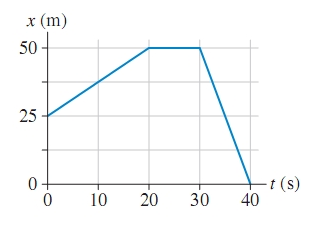# Problem: The figure is the position-versus-time graph of a jogger.A. What is the jogger’s velocity at t = 10 s? B. What is the jogger’s velocity at t = 25 s? C. What is the jogger’s velocity at t = 35 s?

###### FREE Expert Solution

Velocity:

$\overline{){\mathbf{v}}{\mathbf{=}}\frac{\mathbf{∆}\mathbf{x}}{\mathbf{∆}\mathbf{t}}}$

This shows that the slope of the position-versus-time graph gives the velocity of the jogger.

A.

At t = 10s, we'll consider the slope of the first part of the motion between 0s and 20s.

97% (122 ratings)###### Problem Details

The figure is the position-versus-time graph of a jogger.A. What is the jogger’s velocity at t = 10 s?

B. What is the jogger’s velocity at t = 25 s?

C. What is the jogger’s velocity at t = 35 s?